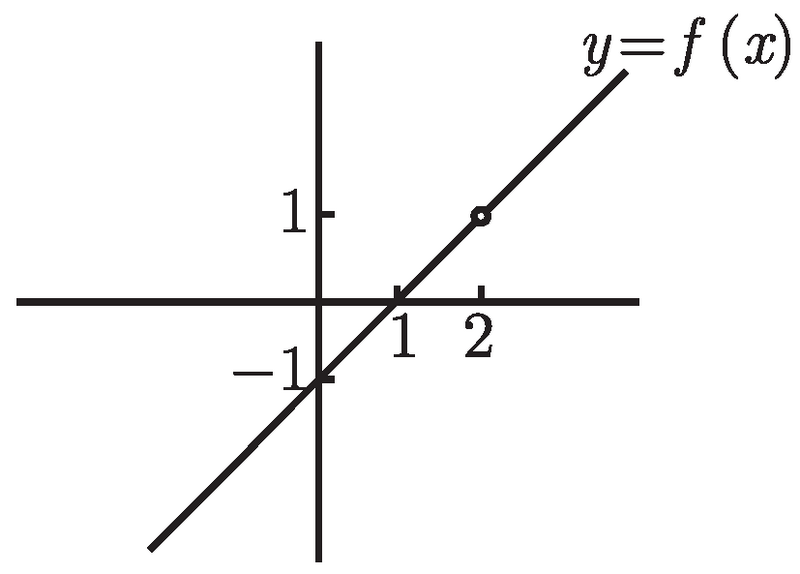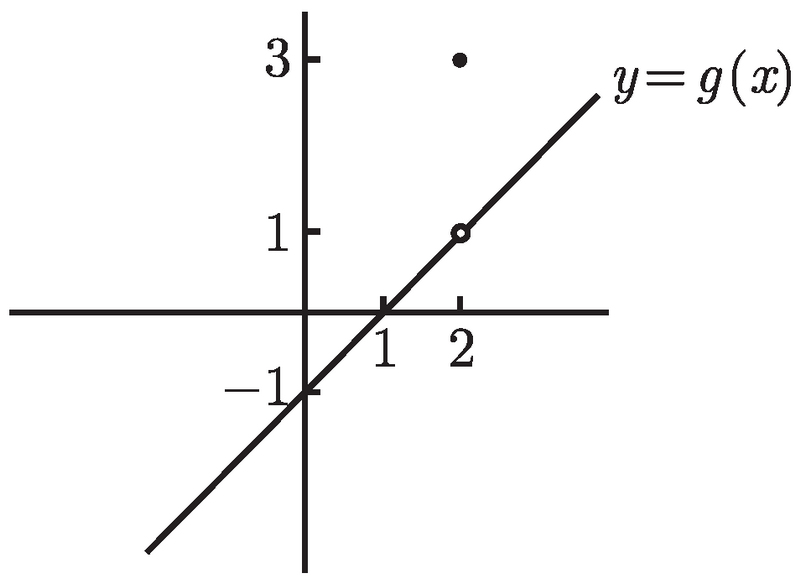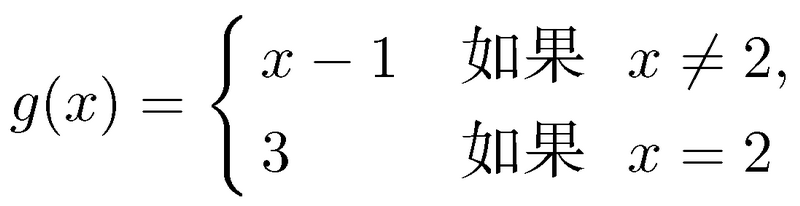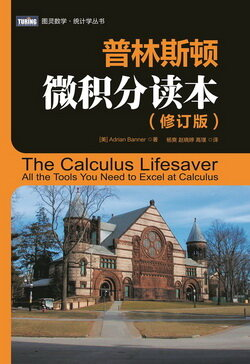# 普林斯顿微积分读本（修订版）(21)：极限导论 3&3.1(极限导论)

• 对于极限是什么的直观概念 ;
• 左、右与双侧极限, 及在 ∞ 和 -∞ 处的极限 ;
• 何时极限不存在 ;
• 三明治定理 (也称作 “夹逼定理”).

(极限：基本思想)

f (x) = x - 1　当 x ≠ 2.$\lim_{x\to2}f(x)=1.$

f (x) → 1 当 x → 2.$\lim_{x\to2}g(x)$ 是什么呢？这里的关键是, g(2) 的值和该极限是不相关的! 只有那些在 x 接近于 2 时的 g(x) 的值, 而不是在 2 处的值, 才是问题的关键. 如果忽略 x = 2, 函数 g 和之前的函数 f 就是完全相同的. 因此, 尽管 g(2) = 3, 我们还是有 $\lim_{x\to2}g(x)=1$.

$\lim_{x\to2}f(x)=1,$

$\lim_{q\to2}f(q)=1.$

$\lim_{b\to2}f(b)=1,\quad\lim_{z\to2}f(z)=1,\quad\lim_{\alpha\to2}f(\alpha)=1,$

$\lim_{x\to2}f(x)=1,$